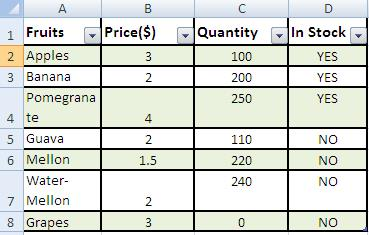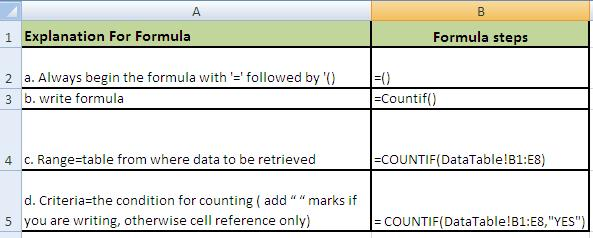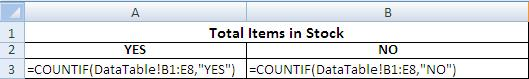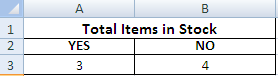# Excel Countif Function

## Step by Step: How to Use Countif in excel 2003, 2007 & 2010

What is Countif? Countif counts data rows if one specified condition is met i.e. If you wish to count how many items are available in store, it will count those items. Remember, don’t confuse Countif with Countifs. Countif is used for a single condition only. However, for more than one condition Countifs is used.

### Step 1: Counting data on the basis of a certain condition in excel

(b) Formulate your tables: these may be on the same table or on a different worksheet or even different workbooks.
(c) For the sake of example we have created two Tables.
(d) Table from where data is to be retrieved:Excel 2007 2010 Countif function

(e) Table where data is to be fetched/desired.Excel Countif function

### Step 2: Excel conditional counting of data

Ensure spellings for search values are the same in both the tables.

### Step 3:MS Excel Count data on criteria basis

Make sure there is no extra space.

### Step 4: MS Excel Counting data on conditionsMS Excel Countif how to

### Final Formula:MS Excel countif Formula

### Result:

Excel Countif FunctionMS excel counting conditional data